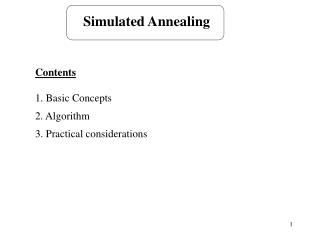# Simulated Annealing - PowerPoint PPT PresentationDownload PresentationSimulated Annealing

Simulated AnnealingDownload Presentation## Simulated Annealing

- - - - - - - - - - - - - - - - - - - - - - - - - - - E N D - - - - - - - - - - - - - - - - - - - - - - - - - - -
##### Presentation Transcript

1. Simulated Annealing Contents 1. Basic Concepts 2. Algorithm 3. Practical considerations

2. Literature 1. Modern Heuristic Techniques for Combinatorial Problems, (Ed) C.Reeves 1995, McGraw-Hill. Chapter 2. 2. Operations Scheduling with Applications in Manufacturing and Services, Michael Pinedo and Xiuli Chao, McGraw Hill, 2000, Chapter 3.6. or Scheduling, Theory, Algorithms, and Systems, Second Addition, Michael Pinedo, Prentice Hall, 2002, Chapter 14.4

3. t    Basic Concepts • Allows moves to inferior solutions in order not to get stuck in a poor local optimum. • c = F(Snew) - F(Sold) F has to be minimised inferior solution (c > 0) still accepted if U is a random number from (0, 1) interval t is a cooling parameter: t is initially high - many moves are accepted t is decreasing - inferior moves are nearly always rejected • As the temperature decreases, the probability of accepting worse moves decreases. c > 0 inferior solution -c < 0

4. Algorithm Step 1. k=1 Select an initial schedule S1 using some heuristic and set Sbest = S1 Select an initial temperature t0 > 0Select a temperature reduction function (t) Step 2. Select ScN(Sk) If F(Sbest) < F(Sc) If F(Sc) < F(Sk) then Sk+1 = Sc else generate a random uniform number Uk If Uk < then Sk+1 = Sc else Sk+1 = Sk else Sbest = Sc Sk+1 = Sc

5. Step 3. tk = (t) k = k+1 ; If stopping condition = true then STOP else go to Step 2

6. Exercise. Consider the following scheduling problem 1 | dj | wjTj . Apply the simulated annealing to the problem starting out with the3, 1, 4, 2 as an initial sequence. Neighbourhood: all schedules that can be obtained throughadjacent pairwise interchanges. Select neighbours within the neigbourhood at random. Choose (t) = 0.9 * t t0 = 0.9 Use the following numbers as random numbers: 0.17, 0.91, ...

7. Sbest= S1 = 3, 1, 4, 2 F(S1) = wjTj = 1·7 + 14·11 + 12·0+ 12 ·25 = 461 = F(Sbest) t0 = 0.9 Sc = 1, 3, 4, 2 F(Sc) = 316 < F(Sbest) Sbest= 1, 3, 4, 2 F(Sbest) = 316 S2 = 1, 3, 4, 2 t = 0.9 · 0.9 = 0.81 Sc = 1, 3, 2, 4 F(Sc) = 340 > F(Sbest) U1 = 0.17 > = 1.35*10-13 S3= 1, 3, 4, 2 t = 0.729

8. Sc = 1, 4, 3, 2 F(Sc) = 319 > F(Sbest) U3 = 0.91 > = 0.016 S4= S4 = 1, 3, 4, 2 t = 0.6561 ...

9. Practical considerations • Initial temperature • must be "high" • acceptance rate: 40%-60% seems to give good results in many situations • Cooling schedule • a number of moves at each temperature • one move at each temperature • t =  ·t  is typically in the interval [0.9, 0.99]  is typically close to 0 • Stopping condition • given number of iterations • no improvement has been obtained for a given number of iteration